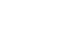Study Guides (390,000)
US (220,000)
KSU (400)
BUS (10)
Final

# BUS 10123 Chapter Notes - Chapter 13: Autoregressive Conditional Heteroskedasticity, Stock Market Index, HeteroscedasticityExam

Department
Course Code
BUS 10123
Professor
Eric Von Hendrix
Study Guide
Final

This preview shows pages 1-2. to view the full 7 pages of the document.1
1. (a) The scope of possible answers to this part of the question is limited only
by the imagination! Simulations studies are useful in any situation where the
conditions used need to be fully under the control of the researcher (so that
an application to real data will not do) and where an analytical solution to the
problem is also unavailable. In econometrics, simulations are particularly
useful for examining the impact of model mis-specification on the properties
of estimators and forecasts. For example, what is the impact of ignored
structural breaks in a series upon GARCH model estimation and forecasting?
What is the impact of several very large outliers occurring one after another
on tests for ARCH? In finance, an obvious application of simulations, as well
as those discussed in Chapter 11, is to producing “scenarios” for stress-
testing risk measurement models. For example, what would be the impact on
bank portfolio volatility if the correlations between European stock indices
rose to one? What would be the impact on the price discovery process or on
market volatility if the number and size of index funds increased
substantially?
(b) Pure simulation involves the construction of an entirely new dataset made
from artificially constructed data, while bootstrapping involves resampling
with replacement from a set of actual data.
Which technique of the two is the more appropriate would obviously depend
on the situation at hand. Pure simulation is more useful when it is necessary
to work in a completely controlled environment. For example, when
examining the effect of a particular mis-specification on the behaviour of
hypothesis tests, it would be inadvisable to use bootstrapping, because of
course the boostrapped samples could contain other forms of mis-
specification. Consider an examination of the effect of autocorrelation on the
power of the regression F-test. Use of bootstrapped data may be
inappropriate because it violates one or more other assumptions for
example, the data may be heteroscedastic or non-normal as well. If the
bootstrap were used in this case, the result would be a test of the effect of
several mis-specifications on the F-test!
Bootstrapping is useful, however, when it is desirable to mimic some of the
distributional properties of actual data series, even if we are not sure quite
what they are. For example, when simulating future possible paths for price
series as inputs to risk management models or option prices, bootstrapping is
useful. In such instances, pure simulation would be less appropriate since it

Only pages 1-2 are available for preview. Some parts have been intentionally blurred.2
would bring with it a particular set of assumptions in order to simulate the
data e.g. that returns are normally distributed. To the extent that these
assumptions are not supported by the real data, the simulated option price
or risk assessment could be inaccurate.
(c) Variance reduction techniques aim to reduce Monte Carlo sampling error.
In other words, they seek to reduce the variability in the estimates of the
quantity of interest across different experiments, rather like reducing the
standard errors in a regression model. This either makes Monte Carlo
simulation more accurate for a given number of replications, making the
answers more robust, or it enables the same level of accuracy to be achieved
using a considerably smaller number of replications. The two techniques that
were discussed in Chapter 11 were antithetic variates and control variates.
Mathematical details were given in the chapter and will therefore not be
repeated here.
Antithetic variates try to ensure that more of the probability space is covered
by taking the opposite (usually the negative) of the selected random draws,
and using those as another set of draws to compute the required statistics.
Control variates use the known analytical solutions to a similar problem to
improve accuracy. Obviously, the success of this latter technique will depend
on how close the analytical problem is to the actual one under study. If the
two are almost unrelated, the reduction in Monte Carlo sampling variation
will be negligible or even negative (i.e. the variance will be higher than if
control variates were not used).
(d) Almost all statistical analysis is based on “central limit theorems” and
“laws of large numbers”. These are used to analytically determine how an
estimator will behave as the sample tends to infinity, although the behaviour
could be quite different for small samples. If a sample of actual data that is
too small is used, there is a high probability that the sample will not be
representative of the population as a whole. As the sample size is increased,
the probability of obtaining a sample that is unrepresentative of the
population is reduced. Exactly the same logic can be applied to the number of
replications employed in a Monte Carlo study. If too small a number of
replications is used, it is possible that “odd” combinations of random number
draws will lead to results that do not accurately reflect the data generating
process. This is increasingly unlikely to happen as the number of replications
is increased. Put another way, the whole probability space will gradually be
appropriately covered as the number of replications is increased.